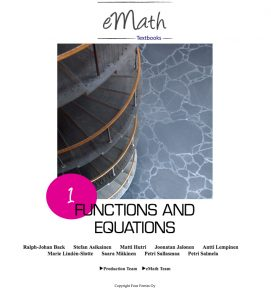# eMath Textbooks

The eMath textbooks are interactive mathematics books. All textbooks use structured derivations as the basic presentation format. The books cover all the central topics in high school mathematics (grades 10 -12). The eMath textbooks are organised according to the Finnish national curriculum for high school mathematics at advanced level.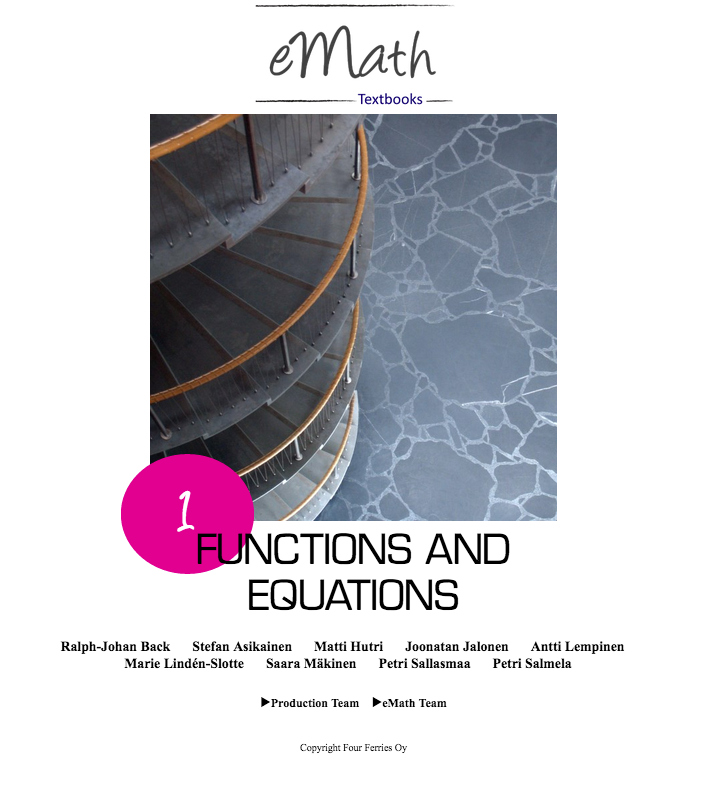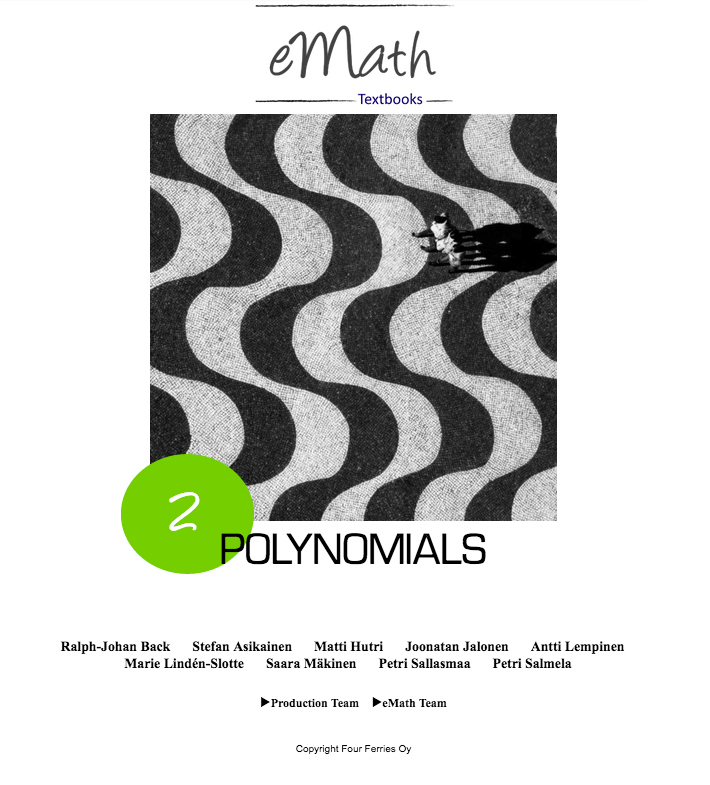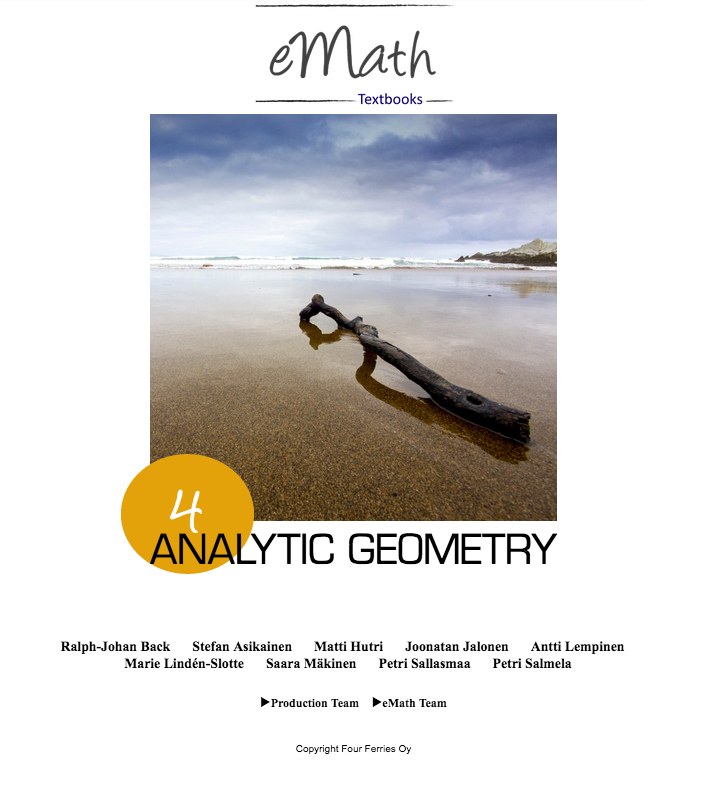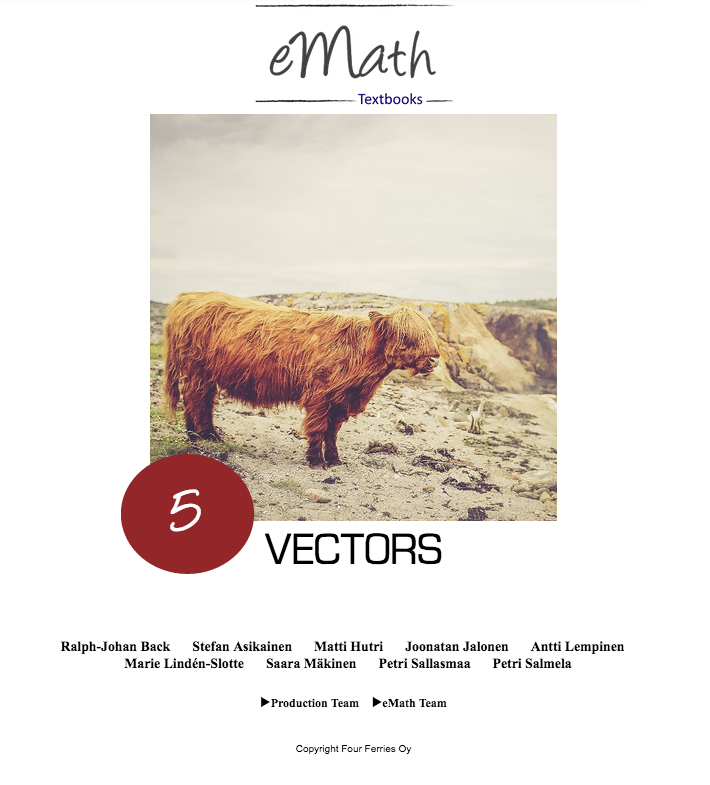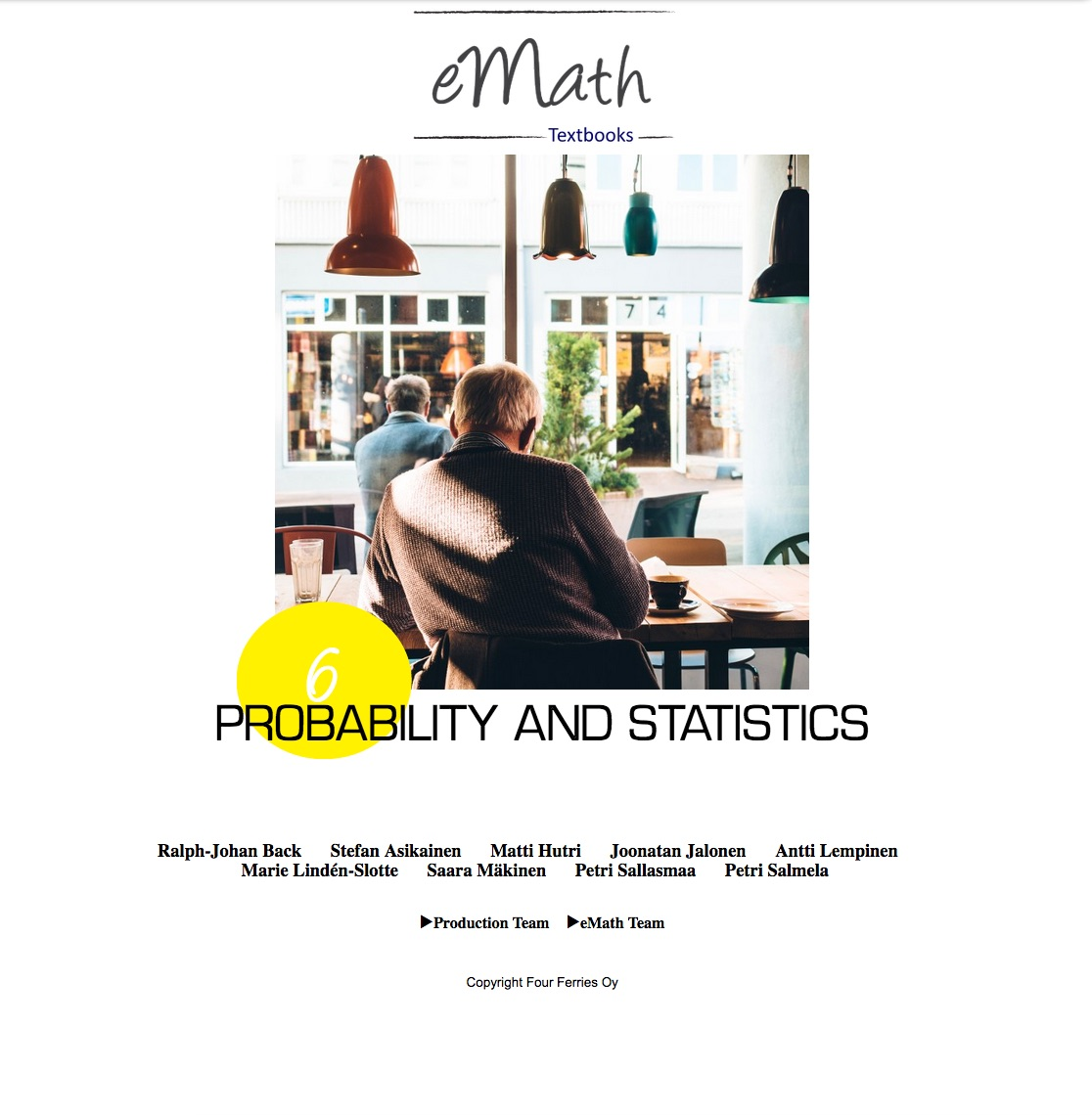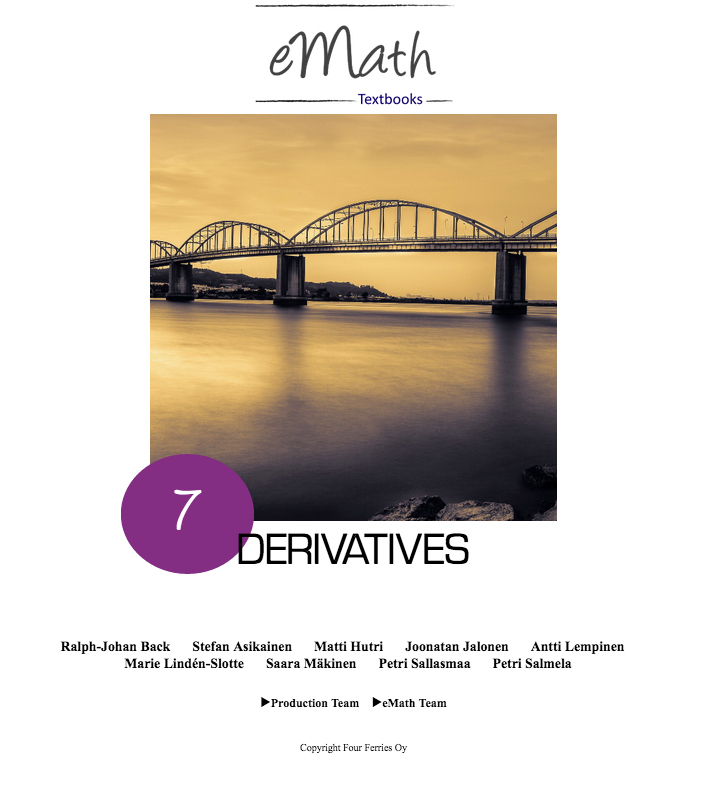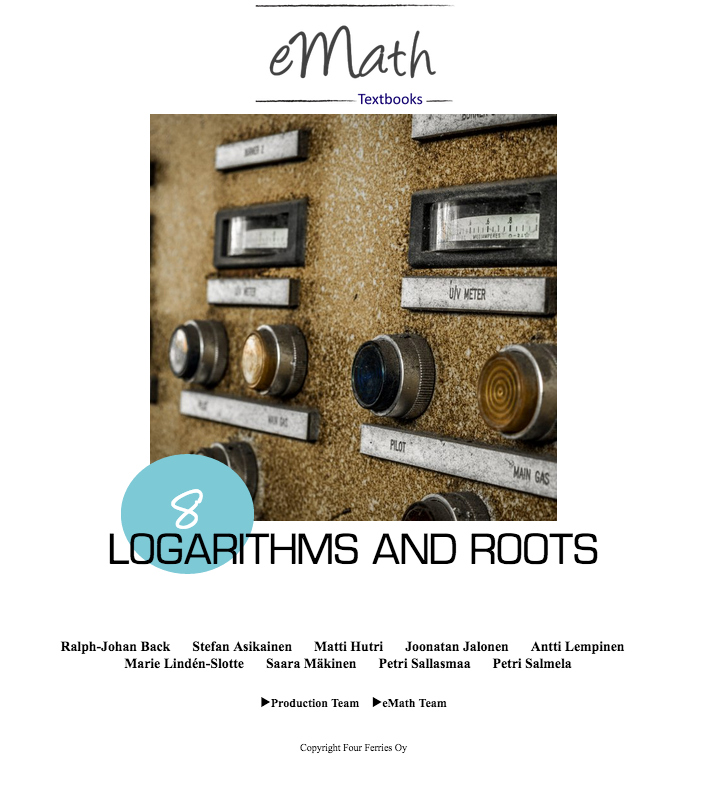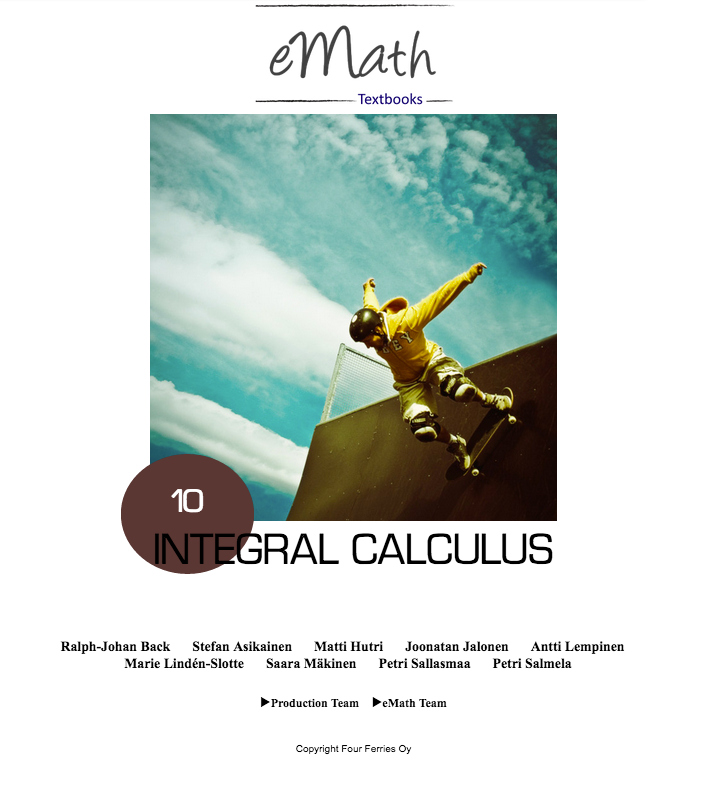### Textbooks Available in English

The books we offer in English cover all areas of high school mathematics:

• eMath 1 Functions And Equations
• eMath 2 Polynomials
• eMath 3 Geometry
• eMath 4 Analytic Geometry
• eMath 5 Vectors
• eMath 6 Statistics And Probability
• eMath 7 Derivatives
• eMath 8 Logarithms and Roots
• eMath 9 Trigonometric Functions and Sequences
• eMath 10 Integrals
This book series is also available by request in Finnish and Swedish.

### Textbooks available in Finnish and Swedish

We offer all the mandatory courses according to the Finnish long mathematics curriculum (2016):

• eMath MAY1 Numbers And Sequences
• eMath MAA2 Polynomial Functions And Equations
• eMath MAA3 Geometry
• eMath MAA4 Vectors
• eMath MAA5 Analytic Geometry
• eMath MAA6 Derivatives
• eMath MAA7 Trigonometric Functions
• eMath MAA8 Radical And Logarithmic Functions
• eMath MAA9 Integral Calculus
• eMath MAA10 Propability And Statistics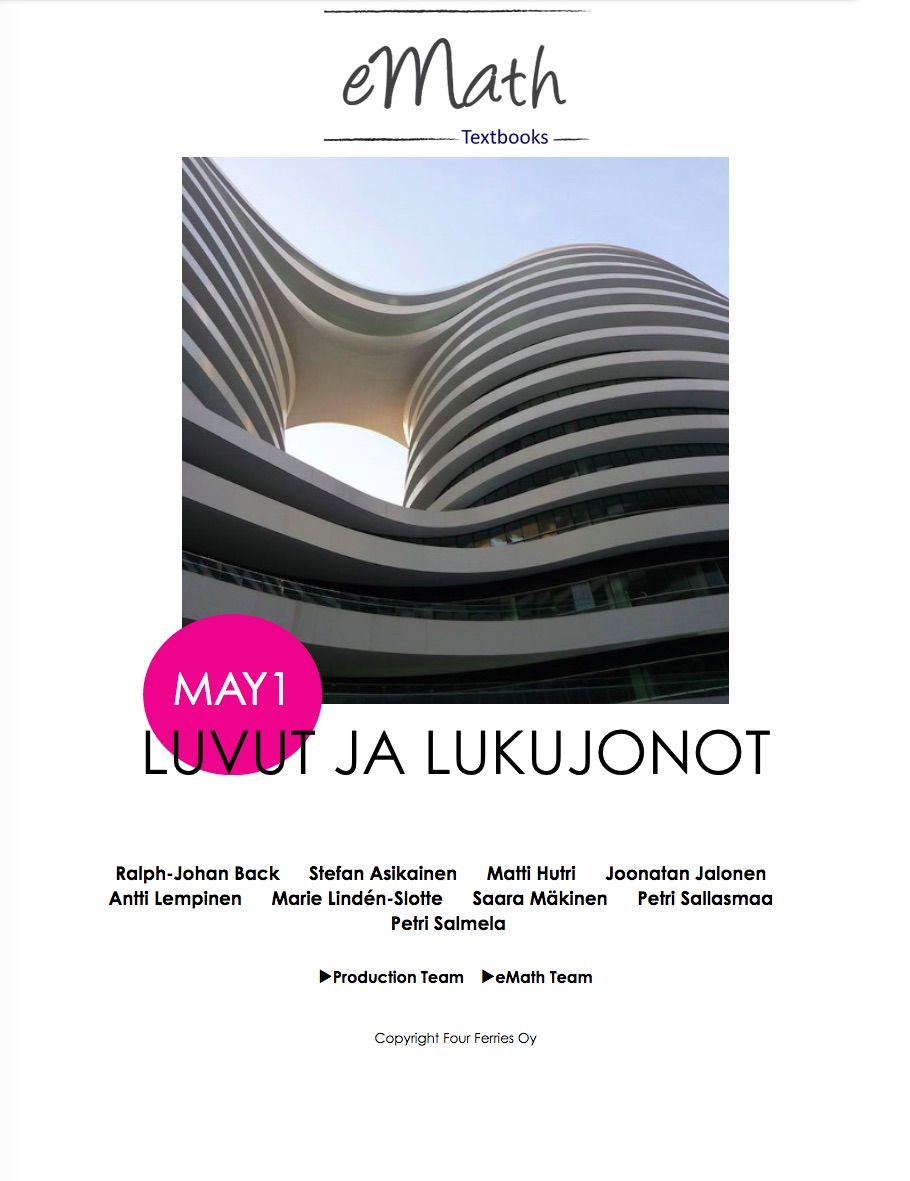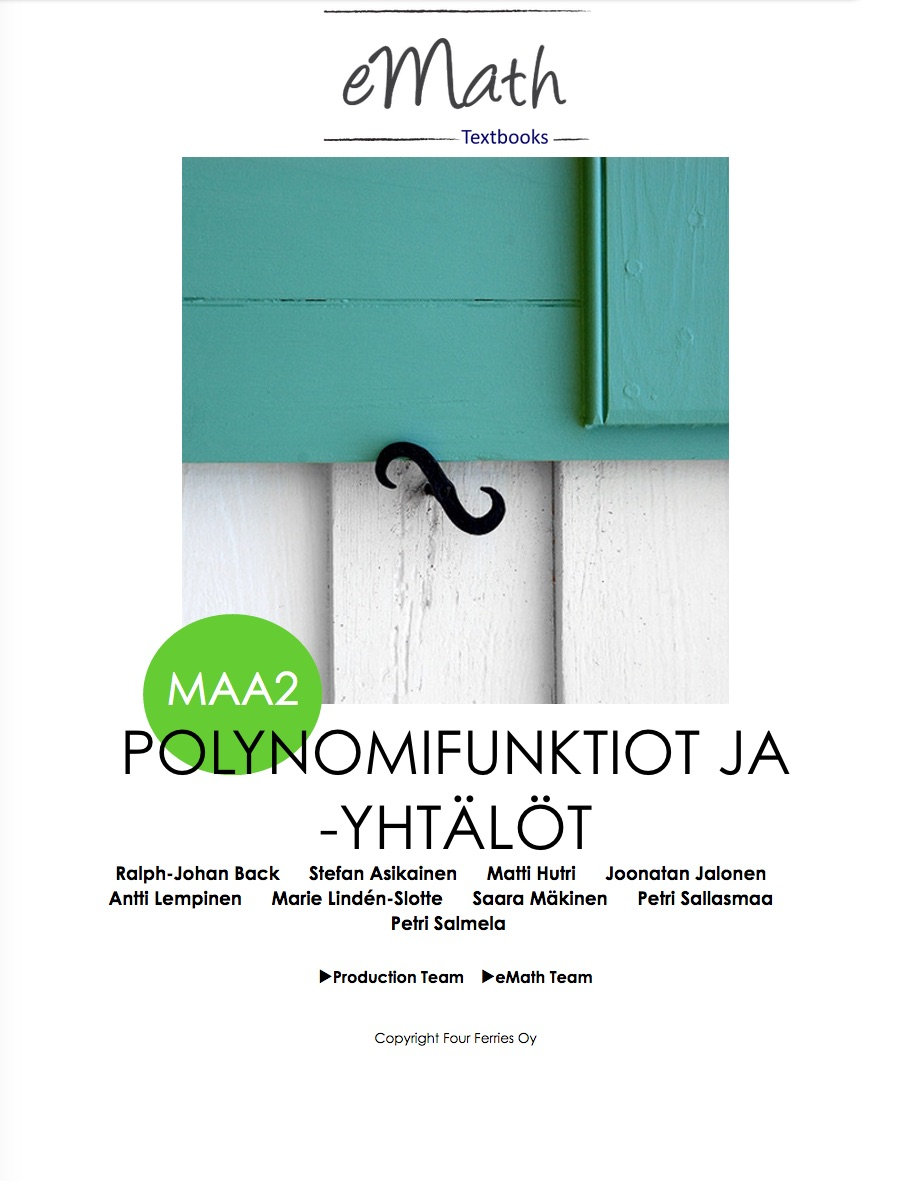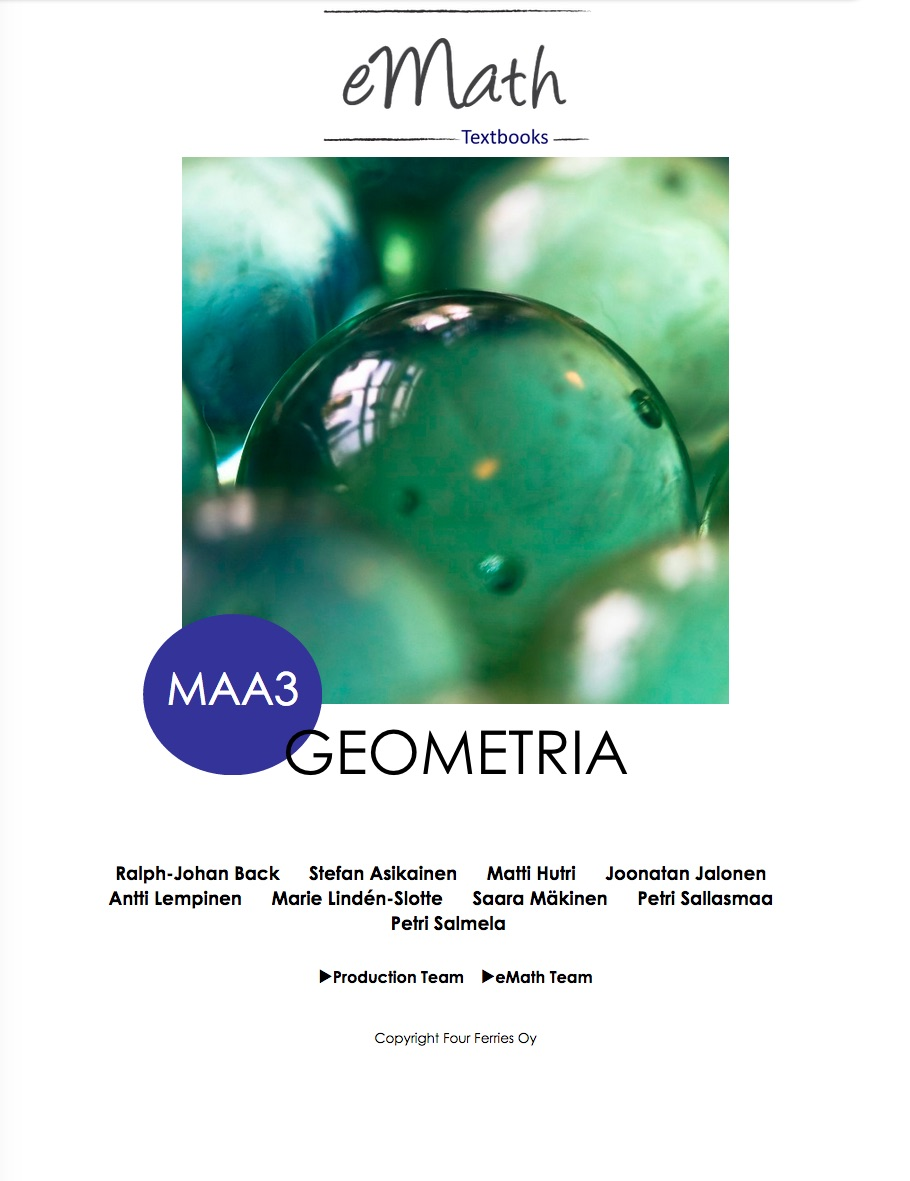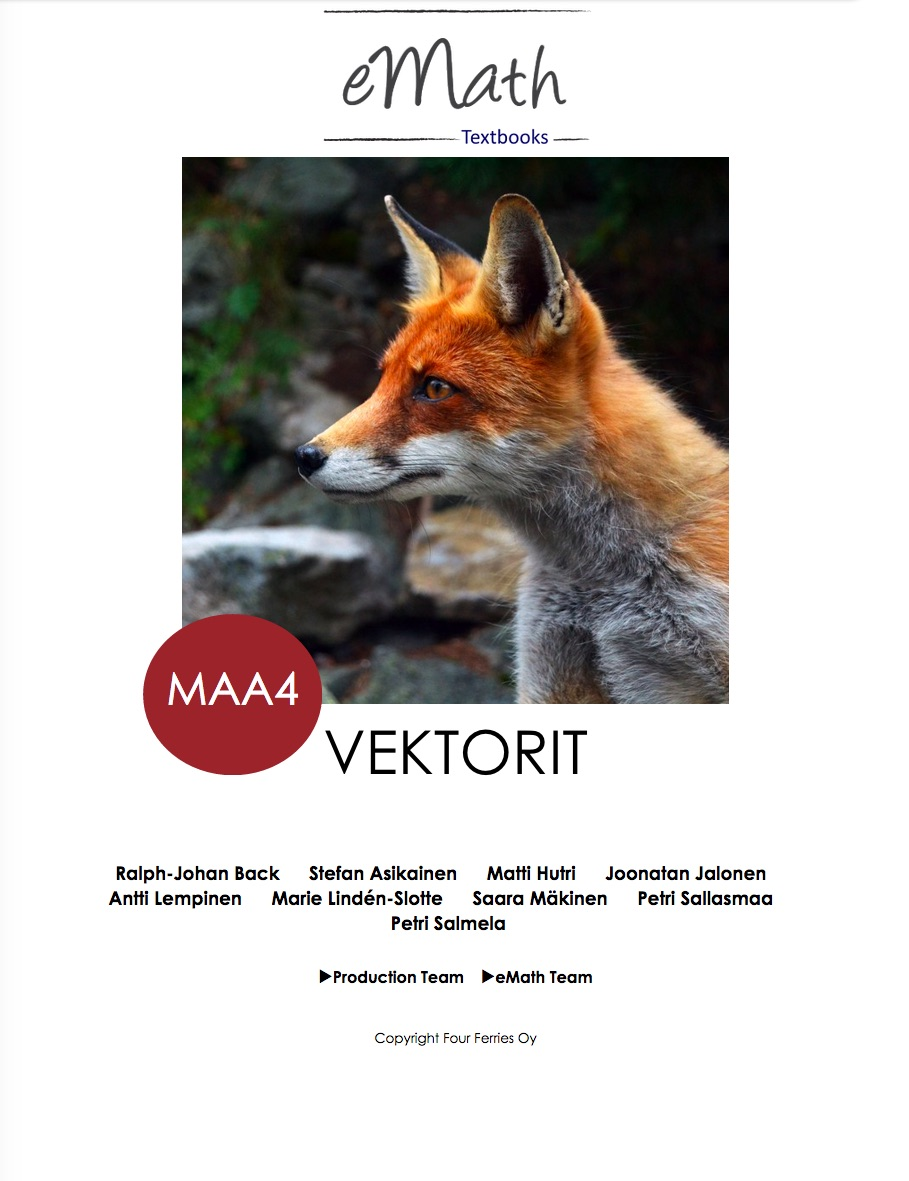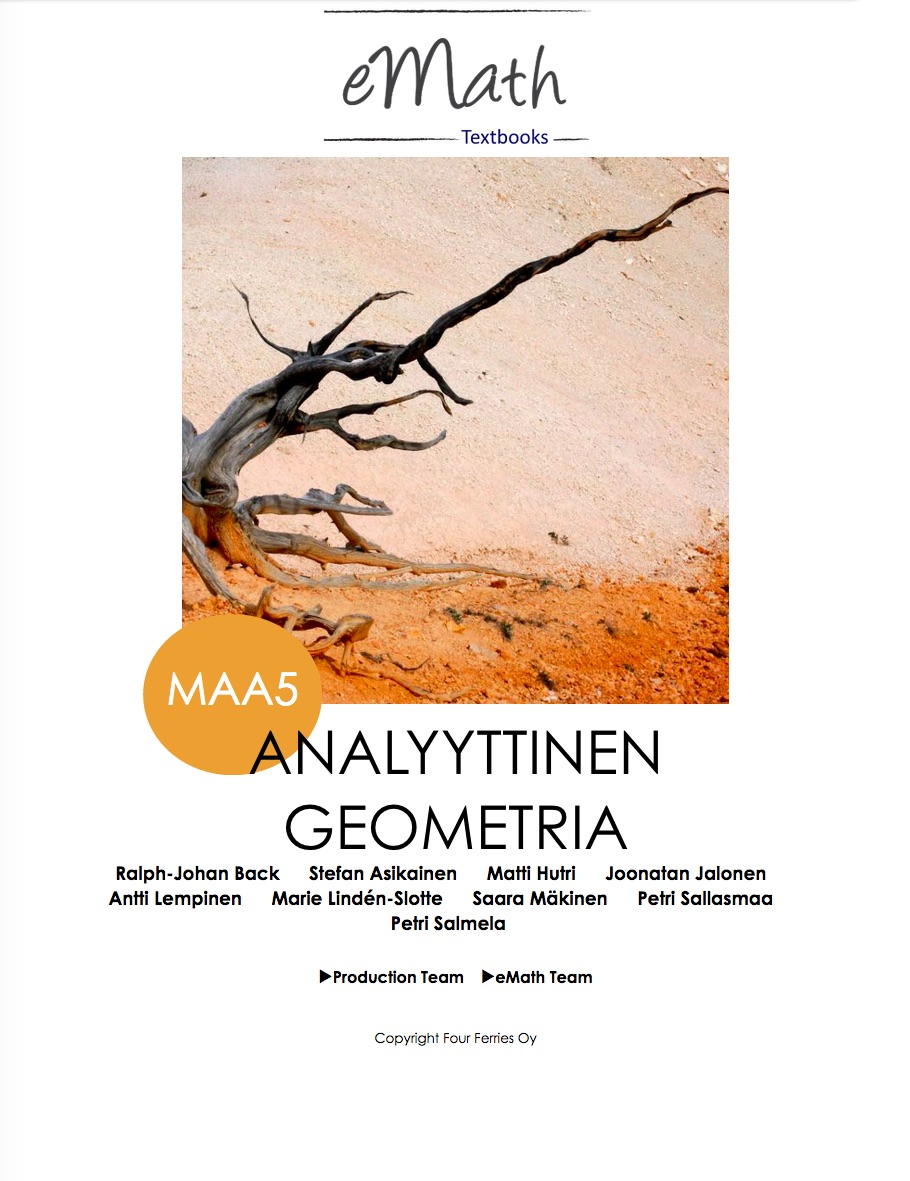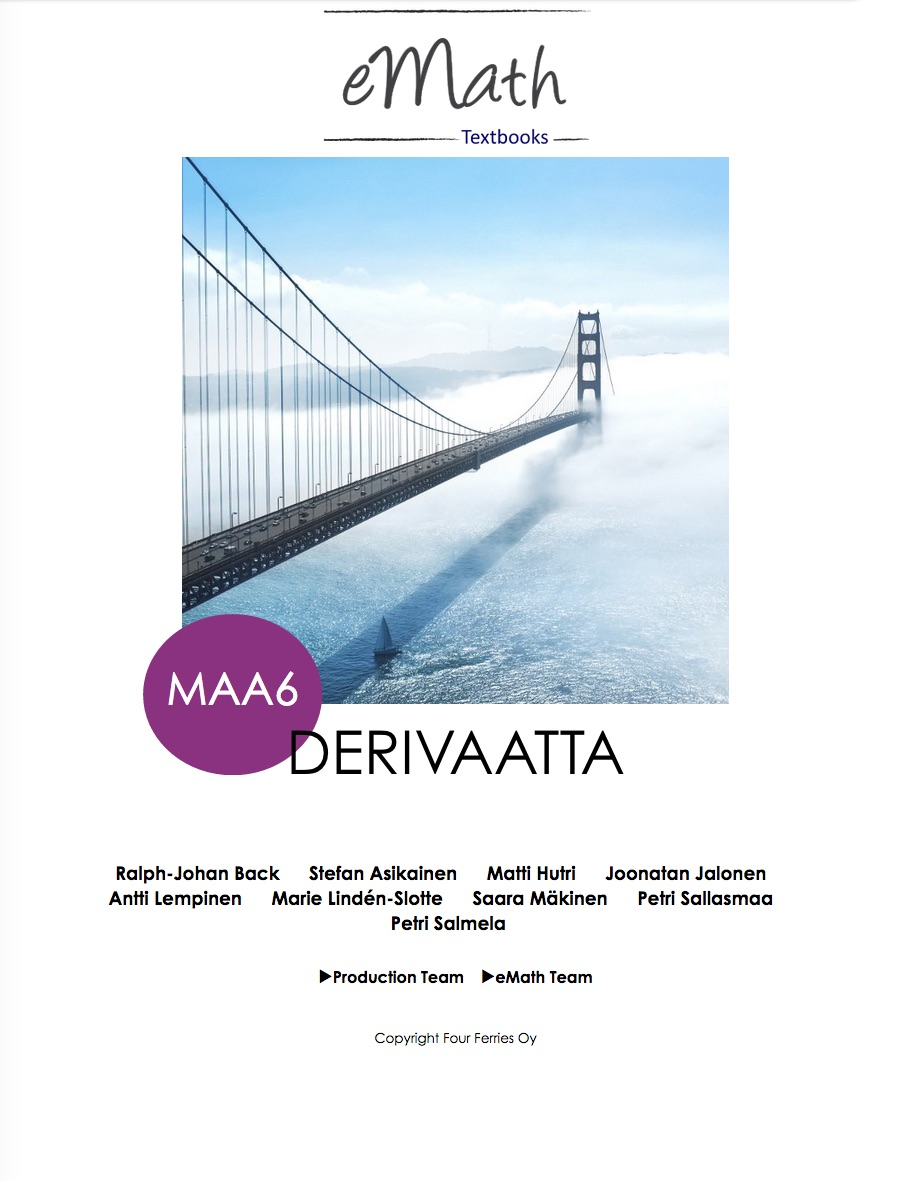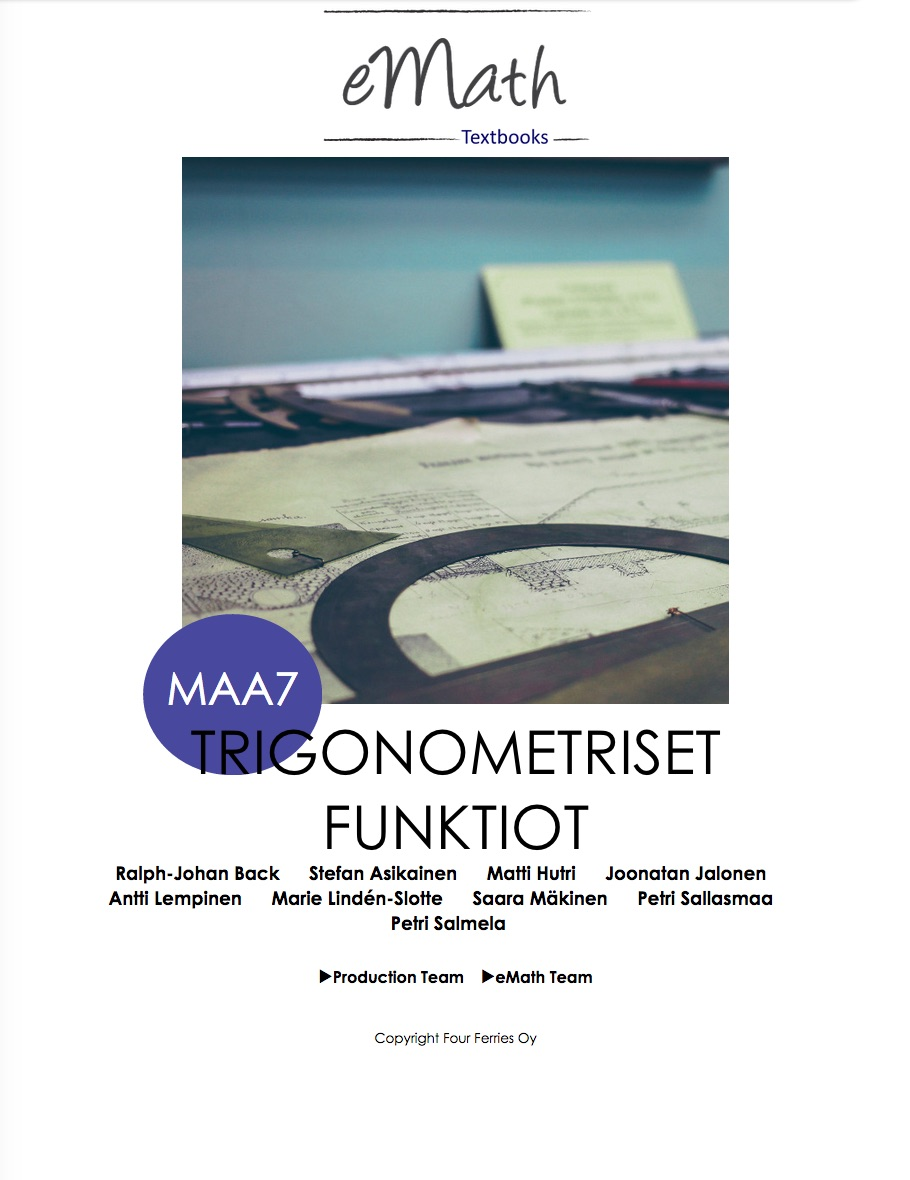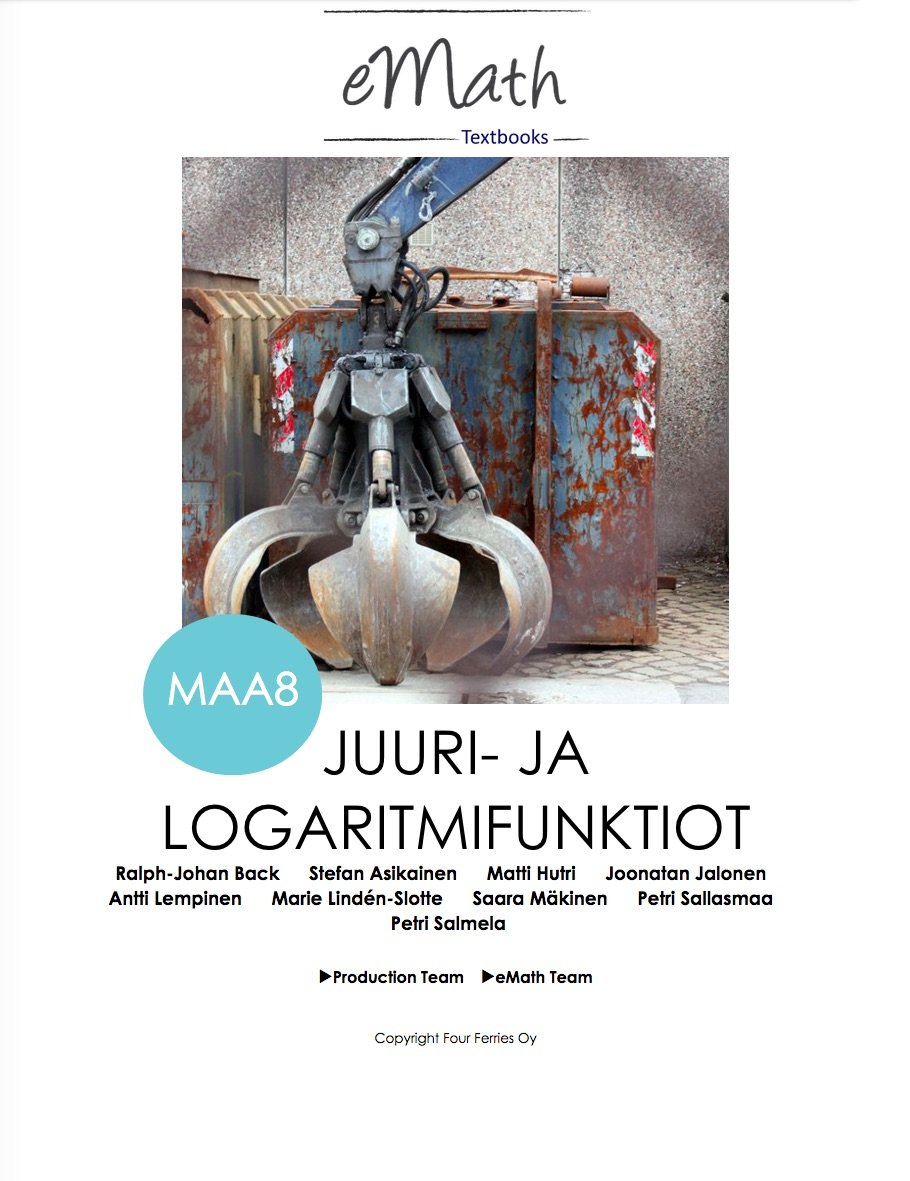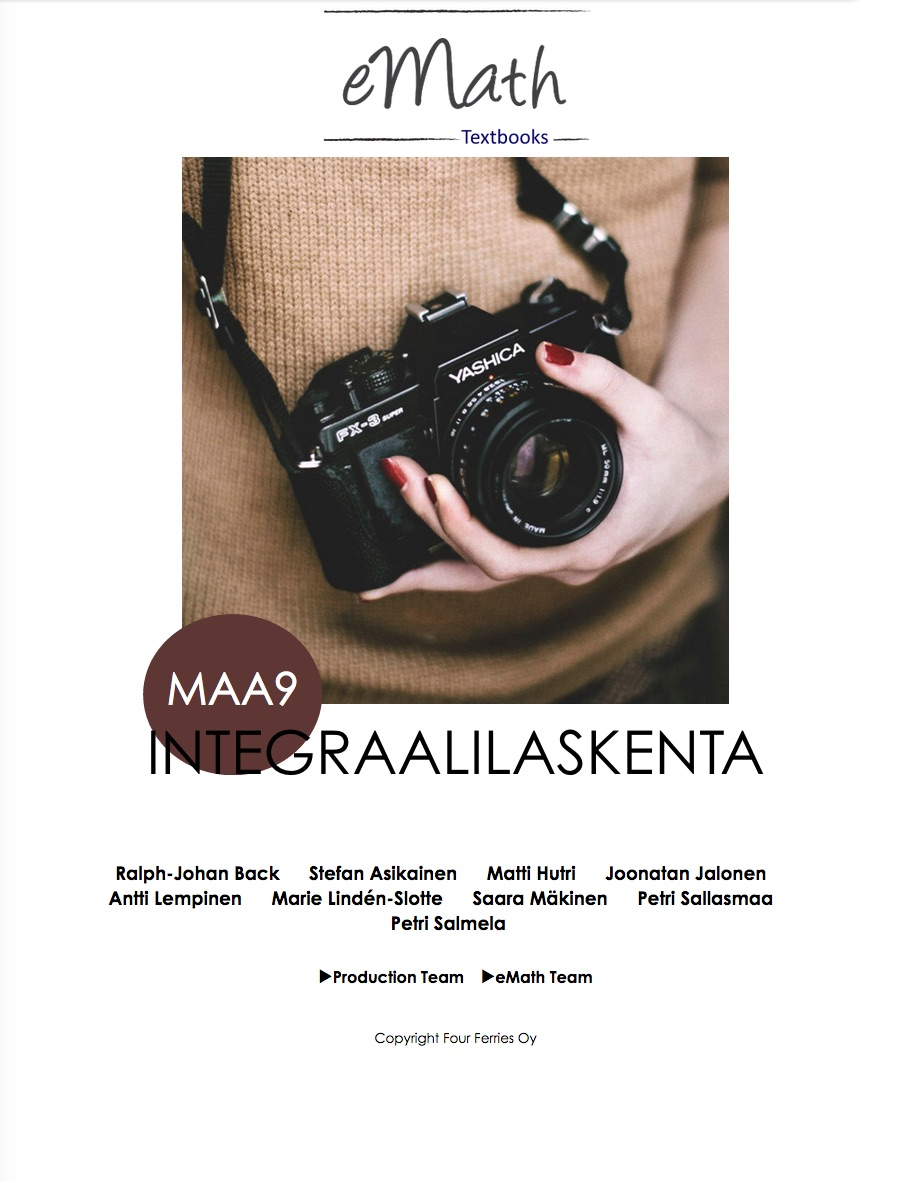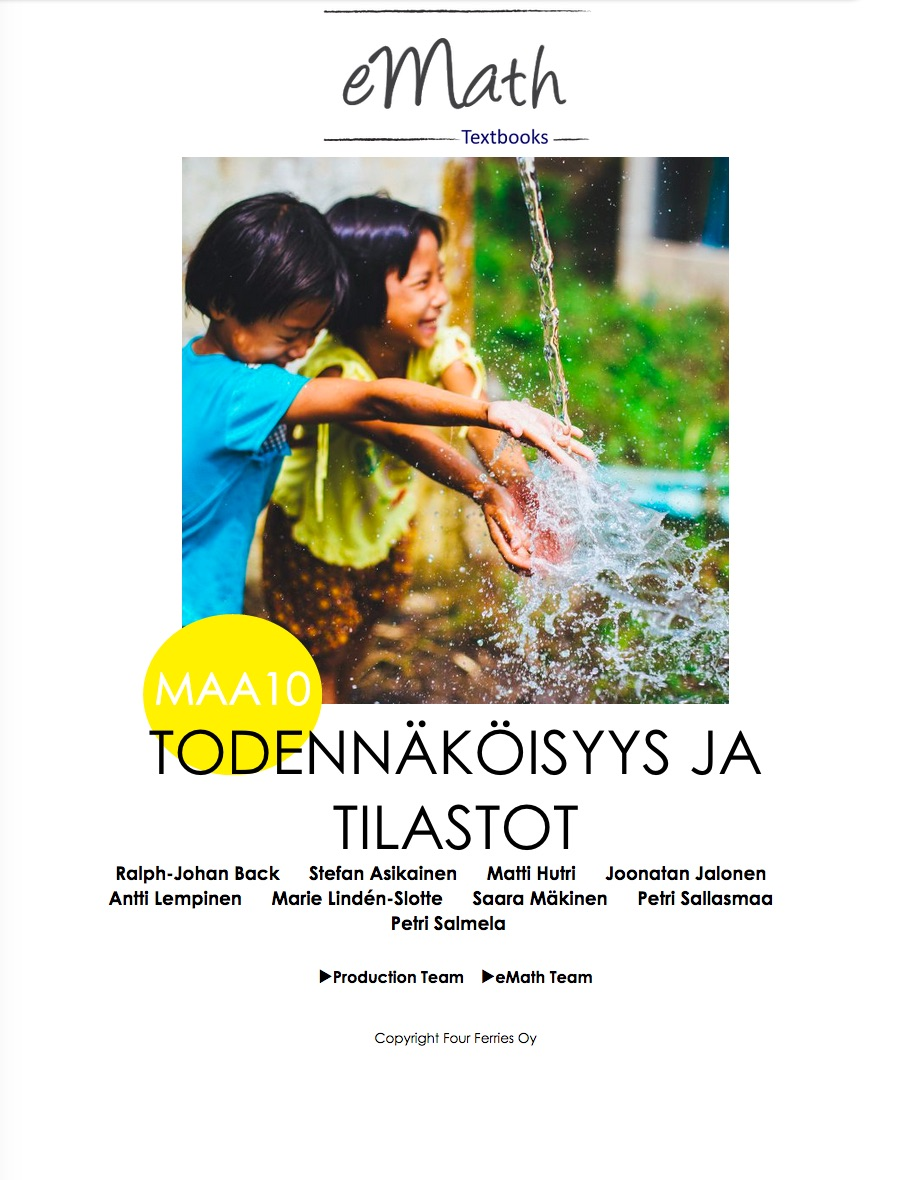The first textbook in the eMath series, eMath 1, can be downloaded for free for iPad tablets.